## Volume 6 - Issue 3

Research Article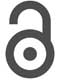Creative Commons, CC-BY

# Useful Generalization of the Inverse Lomax Distribution: Statistical Properties and Application to Lifetime Data

*Corresponding author: Obubu Maxwell, Department of Statistics, Nnamdi Azikiwe University, Awka, Nigeria

Received: November 15, 2019; Published: November 26, 2019;

#### Abstract

A four-parameter compound continuous distribution for modeling lifetime data known as the Odd Generalized Exponentiated Inverse Lomax distribution was proposed in this study. Basic Structural properties were derived in minute details. Simulation studies was carried out to investigate the behavior of the proposed distribution, from which the maximum likelihood estimates for the true parameters, including the bias and root mean square error (RMSE) were obtained. The proposed model was applied to the strengths of glass fibres dataset and the result compared to other existing distribution.

Keywords: Odd generalized exponentiated family, Inverse lomax distribution, Moments, Reliability function, Hazard function, Continuous distribution

#### Introduction

Various distributions have been proposed to serve as models for wide applications on data from different real-life situations through the extension of existing distribution. This has been achieved in various ways. The Lomax distribution also called “Pareto type II” is a special case of the generalized beta distribution of the second kind , and can be seen in many application areas, such as actuarial science, economics, biological sciences, engineering, lifetime and reliability modeling and so on . This heavy-duty distribution is considered useful as an alternative distribution to survival problems and life-testing in engineering and survival analysis . Inverse Lomax distribution is a member of the inverted family of distributions and discovered to be very flexible in analyzing situations with a realized non-monotonic failure rate .If a random variable Χ has a Lomax distribution, thenhas an inverse Lomax Distribution. Thus, a random variable X is said to have an In verted Lomax distribution if the corresponding probability density function and cumulative density function are given by :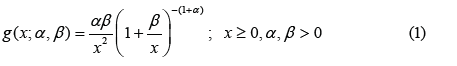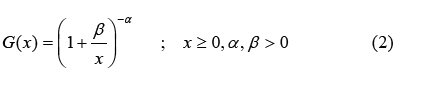In literature exist several families of distribution and the references to them are listed in [6, 7]. In the course of this study, the Odd Generalized Exponential family of distribution developed by 8 having probability density and cumulative distribution function given by;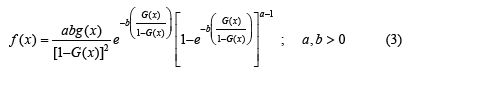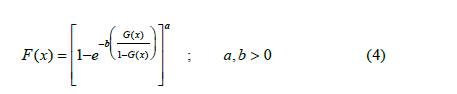was used to extend the Inverse Lomax distribution. The goal is to derive a continuous compound distribution that would be robust and tractable than the Inverse Lomax distribution. The rest of the article is structured as follows: In section 2, we derive the cumulative distribution function, probability density function, reliability function, odd function, hazard function, reverse hazard function, and cumulative hazard function of the Odd Generalized Exponentiated Inverse Lomax distribution, and present their respective plots for different values of the parameter. In minute details, we establish the structural properties, which include the asymptotic behavior, moments, quantile function, median, Skewness and kurtosis, in section 3. In section 4, we determined the order statistics and the estimate of the unknown parameter using maximum likelihood estimation techniques. Application to two different lifetime dataset and a conclusion is made in section 5.

#### The Odd Generalized Exponentiated Inverse Lomax (OGE-IL) distribution

A four parameter Odd Generalized Exponentiated Inverse Lomax distribution was investigated in this section. Obtained by substituting equation (2) into (4), the cumulative distribution function of the OGE-IL distribution is given by;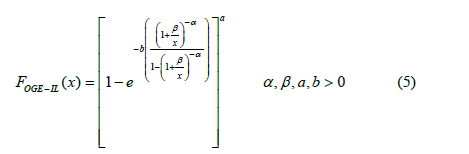The corresponding probability density function is given by;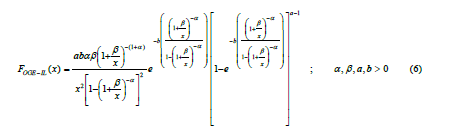The reliability function is given by;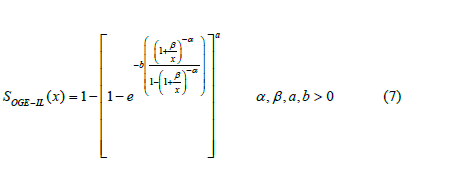The failure rate function/hazard function is given by;The reverse hazard function is given by;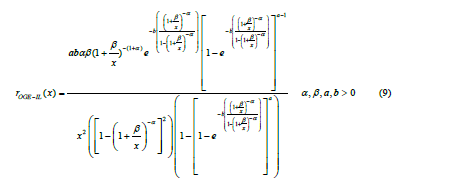The cumulative hazard function is given by;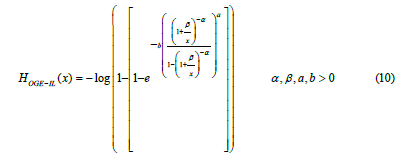And the odd function is given by;The respective plots of the probability density function, cumulative distribution function, reliability function, and the hazard functions for different parameter values are represented in(Figure 1), (Figure 2), (Figure 3)

#### Structural Properties of the Odd Generalized Exponentiated Inverse Lomax (OGE-IL) distribution

In this section we derive some of the basic theoretical/structural properties of the OGE-IL distribution, such as; its asymptotic behavior, quantile, median, Skewness and kurtosis of the Odd Generalized Exponentiated Inverse Lomax distribution.

##### Asymptotic Behavior of the OGE-IL Distribution

We examine the behavior of the OGE-IL distribution in equation (6) as x→0 and as x→∞ and in equation (10) as i.e. x→∞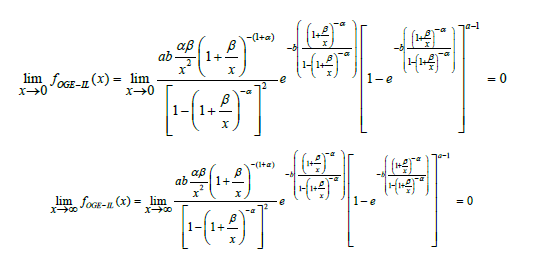Thus showing that the Odd Generalized Exponentiated Inverse Lomax distribution is unimodal i.e. it has only one mode

We can also deduce that for the OGE-IL Distribution,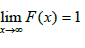i.e.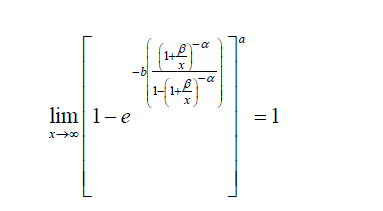Thus, indicating that the OGE-IL distribution has a proper probability density function.

##### Quantile function and Median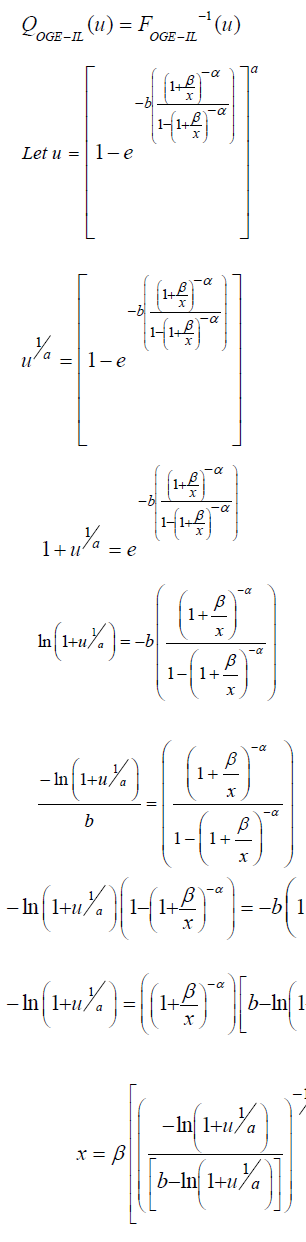The Quantile function of the Odd Generalized Exponentiated Inverse Lomax distribution is;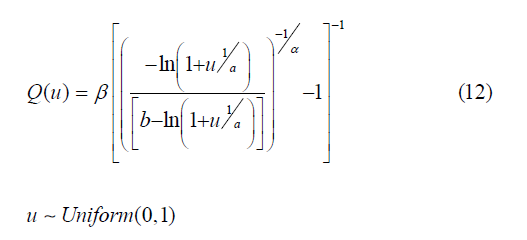The median of the Odd Generalize Exponentiated Inverse Lomax distribution can be gotten by placing u as 0.5 in equation (12) above, we obtain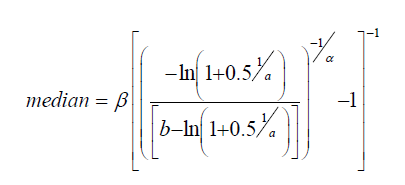##### Skewness and kurtosis

According to Kenney and Keeping , 9, the Bowely Quartile Skewness is given as;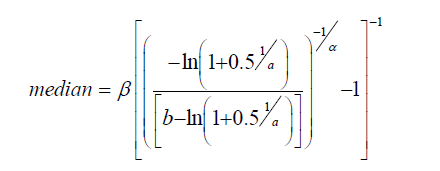Moors quantile-based Kurtosis by Moors , 10 is given as;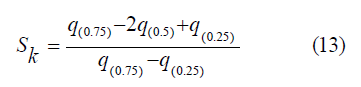##### MomentUsing binomial expansion and solving through, we obtained an expression for the moment as;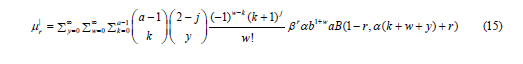#### Order Statistics

Consider random sample denoted byfrom the densities of the Odd Generalized Exponentiated Inverse Lomax distribution. Then,The pdf of the jth order statistics for the OGE-IL distribution is given as;The minimum order statistics for the OGE-IL distribution is given as;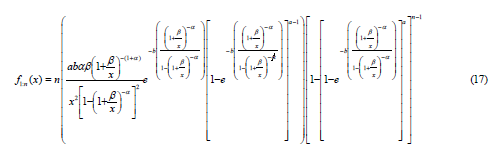And the maximum order statistics for the OGE-IL distribution is given as;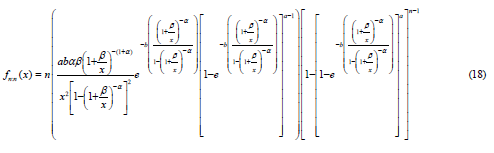#### Parameter Estimation

Using maximum likelihood estimation techniques, let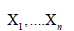indicate a random sample of the complete OGE-ILD data, and then the sample’s likelihood function is given as;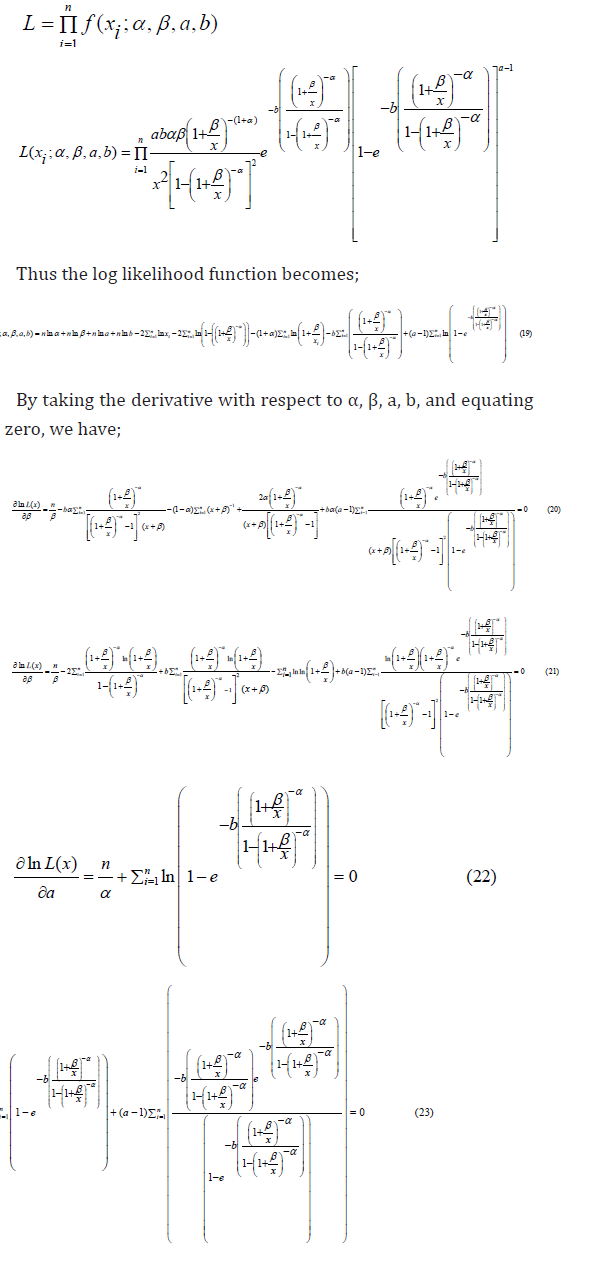The parameters of the OGE-IL distribution is obtained by solving equation 20-24.

#### Simulation

In simulation studies using R software, the behavior of the OGE-IL distribution parameters was investigated. Data sets from the OGE-IL distribution were generated with replication number m=1000, random samples were selected in sizes 50, 100, 200, and 300. The simulation was performed with different true parameter values for four different cases. The selected true parameter values are, α=0.5, β=0.5, a=0.5, and b=0.5; α=1.0, β=1.0, a=1.0, and b=1.0; α=2.0, β=2.0, a=2.0, and b=2.0; α=3.0, β=3.0, a=3.0, and b=3.0 for the first, second, third, and fourth cases, respectively. Maximum likelihood estimates for the true parameters, including the Bias and Root Mean Square Error (RMSE) were obtained. The results of the simulation study are displayed in (Table 1), (Table 2), (Table 3), (Table 4)below.

Tables 1-4 clearly indicate that RMSE decreases when the sample size increases for all selected parameter values. In addition, the variations of the predictions are closer to the actual parameter values, with the increase in the sample size decreasing overall bias. Thus, the estimates tend approaches the true parameter value, as the sample size increases.

#### Application

The Odd Generalized Exponentiated Inverse Lomax distribution was applied to a real-life dataset and its performance was compared to the Odd Generalized Exponentiated Exponential Distribution (OGE-E) [10, 11, 12], and the Exponential Exponentiated Distribution. The most suitable selection criteria were based on the values of the Log-likelihood, and, Akaike Information Criterion (AIC),

##### Data I: Strengths of Glass Fibres Dataset

The dataset obtained from Smith and Naylor [13, 14, 15, 16] represents the strengths of 1.5 cm glass fibres, measured at the National Physical Laboratory, England. The observations are as follows;

0.55, 0.93, 1.25, 1.36, 1.49, 1.52, 1.58, 1.61, 1.64, 1.68, 1.73, 1.81, 2.00, 0.74, 1.04, 1.27, 1.39, 1.49, 1.53, 1.59, 1.61, 1.66, 1.68, 1.76, 1.82, 2.01, 0.77, 1.11, 1.28, 1.42, 1.50, 1.54, 1.60, 1.62, 1.66, 1.69, 1.76, 1.84, 2.24, 0.81, 1.13, 1.29, 1.48, 1.50, 1.55, 1.61, 1.62, 1.66, 1.70, 1.77, 1.84, 0.84, 1.24, 1.30, 1.48, 1.51, 1.55, 1.61, 1.63, 1.67, 1.70, 1.78, 1.89.(Table 5), (Table 5)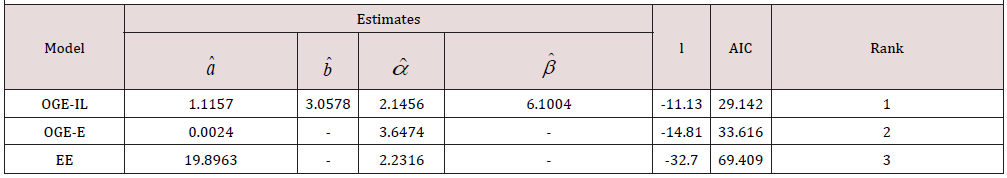Table 6: Summarized results of fitting different distributions to strength of glass fibre dataset.

#### Conclusion

Using the Odd Generalized Exponential family of distribution, we have successfully extended the Inverse Lomax distribution from a two parameter to a four-parameter distribution. Densities and theoretical characteristics for the proposed OGE-IL distribution have been derived. Simulation studies reveal that the root mean square error decreases for the selected parameter values when the sample size increases. The proposed distribution was applied to a lifetime dataset and compared to other existing distribution which it outperformed based on log-likelihood and AIC values.

None

#### Conflict of Interest

None